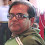## Friday, February 05, 2010

### ALTERNATIVE PHYSICS

SALIENT TESTABLE PREDICTIONS CONTAINED IN OUR
FORTHCOMING BOOK.
The following are a few salient testable predictions contained in this book:
1. The accepted value of the electric charge of quarks contains an error element of 3%. In stead of +⅔ and -⅓, it should be +7/11 and -4/11. Thus, taking the measured charge of electrons as the unit, the value of the electric charge of protons is +10/11 and that of neutrons -1/11. The residual negative charge is not apparent as negative charge always confines positive charge and flows towards the concentration of positive charge - nucleus. Hence it is not felt outside. It is not revealed in measurement due to the nature of calibration of the measuring instruments. Thus, atoms are not charge neutral, but are slightly negatively charged particles. Only that way they can interact with other particles. The negative charge confines the positive charge (nearly 2000 times), which is released during fission and fusion reactions.
2. The value of the gravitational constant G is not the same for all systems. Just like the value for acceleration due to gravity varies from position to position, the value of G also varies between systems. The difference between these two is that while the ‘g’ is internal to a system that balances and confines the smaller mass within a system to the bigger mass (for example, bodies within the lower atmosphere of Earth, which is a part of the Earth system), the gravitational constant G is related to balancing around a point (barycenter) the interaction between the total mass and energy of two closed systems spread over a fixed distance (balancing the free fall) justifying the inverse square law. Thus, it is different for different systems. There are seven types of external and internal gravitational interaction acting simultaneously on all bodies making a total of 49 categories. This classification is derived from fundamental principles.
3. The values of the so-called universal constants are not really constant, but are determined based on the relative strengths of different forces to attain equilibrium, which appear as constant. Thus, it has different values in different mediums. This is due to the laws of symmetry which is derived from the inertia of restoration (also known as elasticity).
4. The value of the fine-structure constant α that determines the electromagnetic field strength as calculated by us theoretically is 7/960 (1/137) when correlated to the strong interaction (zero energy level) and 7/900 (1/128) when correlated to the weak interaction (80 GeV level). There are 5 more values that determine the structure of the orbitals in the atomic spectra. Hence the physically available values of the s orbitals (principal quantum number) are restricted to n = 7, though according to the modern theory, it can have any positive integer value.
5. All Quantum particles have equivalent macro particles. For example, the internal structure of proton and planet Jupiter are identical. So are other systems.
6. What is an electron? What is its internal structure? What is a photon? These have been derived from fundamental principles and discussed with proof.
7. The “measurement problem” is more a problem of wrong concepts than that of physics. Measurement is a comparison between similars. The so-called collapse of the “wave-function” is real, but has a different interpretation.
8. Uncertainty relation is a natural phenomenon described using wrong mathematics. Causality and determinism are not separate concepts, but are complementary concepts revealed in the equations as cause (free-will) and effect (determinism).
9. Conservation of mass and energy is a universal law. However, as Paul Marmet has shown: general relativity is not compatible with the principle of mass-energy conservation. This has been discussed in detail to derive mass and energy from fundamental principles, establish mass-energy equivalence and conditions for their conversion.

#### 1 comment:

1.WISH YOU VERY BEST IN THE FIELD OF BLOGGING!

let noble thoughts come to us from all around